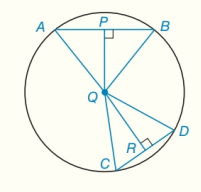Chapter 6.CT, Problem 14CTElementary Geometry For College St...

7th Edition
Alexander + 2 others
ISBN: 9781337614085

Solutions

Chapter
SectionElementary Geometry For College St...

7th Edition
Alexander + 2 others
ISBN: 9781337614085
Textbook Problem

a) If m A B ⌢ > m C D ⌢ , write an inequality that compares m ∠ A Q B       m ∠ C Q D .b) If Q R > Q P , write an inequality that compares A B and C D .To determine

a)

An inequality that compares mAQBandmCQD.

Explanation

Given:

mAB>mCD.

Approach:

“In a circle the measure of a central angle is equal to the measure of the arc that it intercepts”.

Calculation:

Consider the given circle with center Q.

From given it is clear that mAB>mCD.

We know that the measure of a central angle of a circle is equal to the measure of the arc that intercept.

Therefore, mAB is an arc made by the intercepts of an angle mAQB and mCD is an arc made by the intercepts of an angle mCQD

To determine

b)

An inequality that compares AB and CD.

Still sussing out bartleby?

Check out a sample textbook solution.

See a sample solution

The Solution to Your Study Problems

Bartleby provides explanations to thousands of textbook problems written by our experts, many with advanced degrees!

Get Started

In Exercise 25-30, find the rules for the composite functions f g and g f. 25. f(x) = x2 + x + 1; g(x) = x2

Applied Calculus for the Managerial, Life, and Social Sciences: A Brief Approach

Use continuity to evaluate the limit. limx43x22x4

Single Variable Calculus: Early Transcendentals, Volume I

In Problems 9-14, evaluate the functions at the given values of the independent variables.

Mathematical Applications for the Management, Life, and Social Sciences